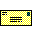# 2022 ARTEMIS SCIENCE NUGGETS

## Spatial scales of the velocity shear layer and Kelvin-Helmholtz waves on the magnetopause: First statistical results.

by Yue Zhou
Zhejiang Provincial Meteorological Bureau of China, Zhejiang, Hangzhou, Shangcheng District, Hehua Chitou, China.

Introduction

Kelvin-Helmholtz (KH) instability typically occurs when a velocity shear exists at the boundary between two layers in a fluid. Its manifestation is KH surface waves or vortices, depending upon the stage in the development of the instability. The solar wind can create a velocity shear along the magnetopause that possibly satisfies the unstable condition for exciting KH waves. Different thicknesses of velocity shear layers and wavelengths of KH waves were observed at different locations of the magnetopause, suggesting that the KH waves may develop when propagating from near-Earth to the magnetotail. In this study, we statistically estimated the thickness of velocity shears that form at the magnetopause before the instability occurs, and the wavelength of KH waves that reach after the instability develops at the magnetopause near Earth and at lunar distance.

Results

 Figure 1. Observations of a KH wave event (left) and a crossing event of velocity shear (right). From top to bottom: the magnetic fields (a, g), ion density (b, h), ion temperature (c, i), ion velocity (d, j), pressure (e, k, thermal pressure in green, magnetic pressure in blue, and total pressure in red), and ion energy flux spectrogram (f, l).

Based on observations from the THEMIS spacecraft, we identified KH wave events at the near-Earth and lunar-distance magnetopause. The left panels of Figure 1 show the observations from ARTEMIS P1 in GSM coordinates collected during the interval 12:00–14:00 UT on 01 May 2018. The quasi-periodic fluctuations of the magnetic field, plasma velocity, and temperature (Figures 1a–c) indicate that the KH waves occurred at the period from 12:28 to 13:23 UT, as marked by the two vertical dotted lines. We estimated the dominant period, phase velocity, and wavelength of the KH waves. A plasma sheet-magnetosheath or low-latitude boundary layer (LLBL)-magnetosheath transition without any signature of KH waves (Figures 1g–i) can be regarded as the initial velocity shear layer, and the right panels of Figure 1 show such an example for 07 September 2017, which possibly develops into KH waves later if an unstable condition is satisfied.

 Figure 2. Relationship between the XGSM location and each of (a) the dominant period, (b) KH wavelength, (c) thickness of velocity shear layers, and (d) the ratio between the KH wavelength and the thickness of velocity shear layers.

Figure 2 shows the distributions of the KH period and wavelength, the thickness of initial velocity shear layer, and their ratio as a function of XGSM, a measure of distance from the center of Earth along the Sun-Earth line. Their dominant period and wavelength at lunar distance are much larger than that on the dayside magnetopause (Figures 2a,b), and the thickness of initial velocity shear layer at lunar distance is similar to that at the near-Earth magnetopause (Figure 2c). It can be seen that the ratio at lunar distance magnetopause ranges from 18 to 41, which is about twice as large as those (7–26) at the near-Earth magnetopause (Figure 2d). According to a linear theory, the range of this ratio is 6–13 for a fastest growing mode. Our result indicates that some of the KH waves observed near Earth are of the fastest growing mode, while the KH waves at lunar distance are not of the fastest growing mode. The factor of two for the ratio is likely related to merging of two vortices.

Conclusion

Using THEMIS observations at the near-Earth magnetopause and ARTEMIS observations at lunar distance, we showed that mean ratio of the wavelength to the thickness of initial shear layers at the lunar distance is twice larger than that for near-Earth. Our result also shows that the range of the ratios estimated at lunar distance is 18–41, which indicates that KH waves were not of the fastest growing mode, that is, already in the stage of full development. The ratio range should be 6–13 according to a linear theory. A statistical study on the combined wavelength and thickness has never been performed before. These results provide some insights into the development of KH waves generated at the dayside and their propagation along the magnetopause to lunar distance.

References

Zhou, Y., Shue, J.-H., Hasegawa, H., Lu, J. Y., Wang, M., & Zhang, H. (2022). Spatial scales of the velocity shear layer and Kelvin-Helmholtz waves on the magnetopause: First statistical results. Geophysical Research Letters, 49, e2021GL097271. https://doi.org/10.1029/2021GL097271

Biographical Note

Yue Zhou was a PhD student of Nanjing University of Information Science &Technology at the time of paper publishing. She is now an assistant researcher at Zhejiang Provincial Meteorological Bureau of China. Her research focuses on Kelvin-Helmholtz instability at the magnetopause.Please send comments/suggestions to
Emmanuel Masongsong / emasongsong @ igpp.ucla.edu